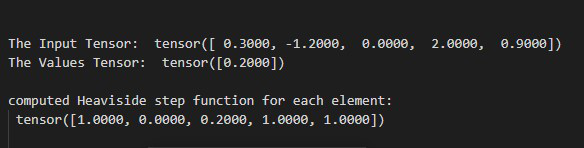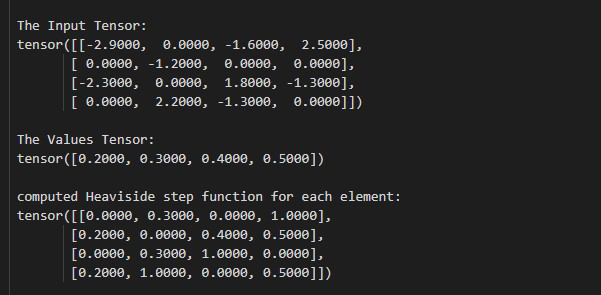# How to Compute the Heaviside Step Function for Each Element in Input in PyTorch?

• Last Updated : 03 Jun, 2022

In this article, we are going to cover how to compute the Heaviside step function for each element in input in PyTorch using Python. We can compute this with the help of torch.heaviside() method.

## torch.heaviside() method

The torch.heaviside() method is used to compute the Heaviside step function for each element. This method accepts input and values as parameters. The parameters type should be tensor only. If the input < 0 then it return 0. whereas, if input > 0 then this method 1 respectively. If the input=0 then this method returns a value the same as the values (one of the parameters). Below is the syntax of the given method:

Syntax: torch.heaviside(input, value)

Parameters:

• input (Tensor): This is our input tensor.
• value (Tensor): This value is a tensor and it’s where input is 0.

Return: This method returns the computed heaviside step function.

### Example 1

In this example, we compute the Heaviside step function for each element in the given 1D tensor.

## Python3

 `# Import the required libraries ` `import` `torch ` ` `  `# define two tensors ` `input_tens ``=` `torch.tensor([``0.3``, ``-``1.2``, ``0``, ``2.0``, ``0.9``]) ` `values_tens ``=` `torch.tensor([``0.2``]) ` ` `  `# display above defined tensors ` `print``(``" The Input Tensor: "``, input_tens) ` `print``(``" The Values Tensor: "``, values_tens) ` ` `  `# compute heaviside step function for each  ` `# element ` `hea ``=` `torch.heaviside(input_tens, values_tens) ` ` `  `# Display Output ` `print``(``" computed Heaviside step function for each element: \n"``, hea) `

Output:### Example 2

In the following example, we compute the Heaviside step function for each element in the given 2D tensor.

## Python3

 `# Import the required libraries ` `import` `torch ` ` `  `# define a 2D tensor for input ` `input_tens ``=` `input` `=` `torch.tensor([[``-``2.9``, ``0.0``, ``-``1.6``, ``2.5``], ` `                                   ``[``0.0``, ``-``1.2``, ``0.0``,  ``0.0``], ` `                                   ``[``-``2.3``, ``0.0``, ``1.8``, ``-``1.3``], ` `                                   ``[``0.0``, ``2.2``, ``-``1.3``, ``0.0``]]) ` ` `  `# define a tensor for values ` `values_tens ``=` `torch.tensor([``0.2``, ``0.3``, ``0.4``, ``0.5``]) ` ` `  `# display above defined tensors ` `print``(``"\n\n The Input Tensor: \n"``, input_tens) ` `print``(``"\n The Values Tensor: \n"``, values_tens) ` ` `  `# compute heaviside step function for each  ` `# element ` `hea ``=` `torch.heaviside(input_tens, values_tens) ` ` `  `# Display Output ` `print``(``"\n computed Heaviside step function for each element: \n"``, hea) `

Output:My Personal Notes arrow_drop_up
Recommended Articles
Page :